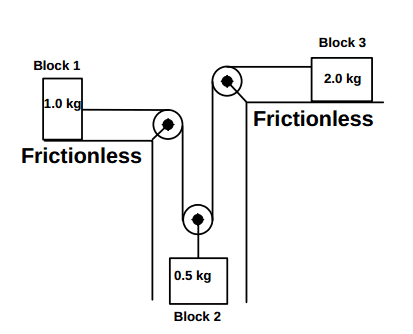# Help with Tension problem

NoobeAtPhysics

## Homework Statement

The diagram shows a block and pulley
system where all the surfaces are
frictionless, and all the pulleys are
massless and frictionless.

Once all Blocks have been let go, what is the ratio of the magnitude of the acceleration, a1, of block 1
to the magnitude of the acceleration of Block 3, a3. That is, what is the ratio a1/a3 ?F=ma

## The Attempt at a Solution

The answer is 2 but I get -2.

On block one FBD
m1 * a1 = T
a=T/m1 = T

FBD on block 3

-T=m3 * a3
a3=-T/m3
a3=-T/2

a1/a3 = -2

Any help would be awesome

## Answers and Replies

Staff Emeritus
Gold Member
First of all, the question asks what the ratio of the MAGNITUDES of the accelerations is. Magnitudes are always positive.

Second, you can't write something like a1 = T/m1 = T. This is nonsense. m1 is not equal to 1.0 (a dimensionless number). It's equal to 1.0 kg, (a physical quantity, with dimensions of mass). You can't write an equation that says acceleration = force. You can't equate two different quantities that have different units. Your equations must be dimensionally consistent. You might think I'm just being picky, but I'm not. It's a fundamental error. You can only equate two physical quantities that have the same dimensions (a force to a force, a mass to a mass, an acceleration to an acceleration, etc.).

•1 person
NoobeAtPhysics
Thanks.

I understand now, magnitude. Sometimes these words pass by without noticing.

also, you're right I probably should write the units. I always do in my written work but I thought everyone would understand what I meant when I shown the work here.

Thank you

Staff Emeritus
Gold Member
Thanks.

I understand now, magnitude. Sometimes these words pass by without noticing.

also, you're right I probably should write the units. I always do in my written work but I thought everyone would understand what I meant when I shown the work here.

Thank you

No problem. I just wanted to point this out because it is a basic error. Just to make sure it's clear: it's not just that you have to include units after you plug in the numbers. It's also your algebraic equation (with only symbols) that I was complaining about. Saying that a = T is actually wrong, and nonsensical (since you're equating an acceleration to a force). You must write a = T/m, even if m = 1.0 kg. You can't just omit the m, because it's a dimensional quantity, it's not just equal to unity. I hope that is clear.

•1 person